# TensorFlow从1到2（八）《从锅炉工到AI专家(6)》一文中，我们把神经网络模型降维，简单的在二维空间中介绍了过拟合和欠拟合的现象和解决方法。但是因为条件所限，在该文中我们只介绍了理论，并没有实际观察现象和应对。

#### 从图中识别过拟合和欠拟合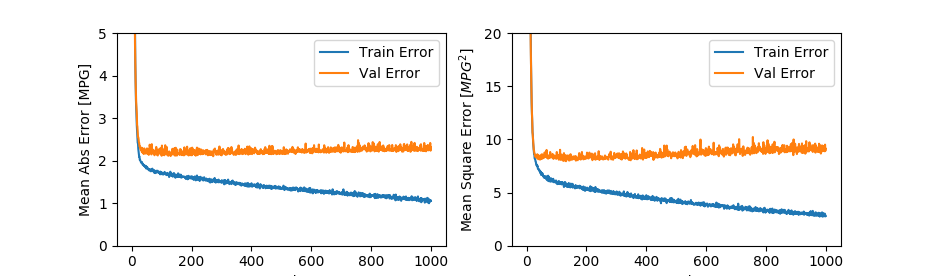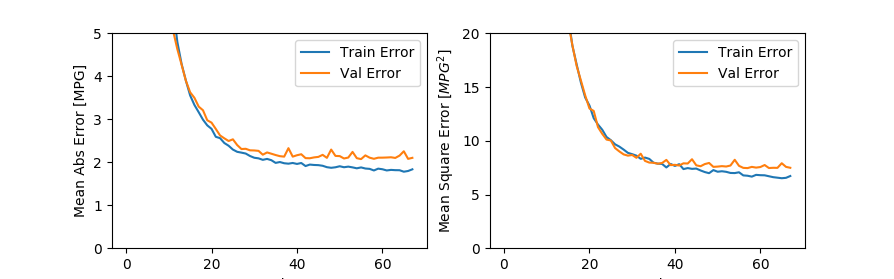先看上边的一组图，随着训练迭代次数的增加，预测的错误率迅速下降。

#### 实验模拟过拟合

• 下载IMDB影评库（仅第一次），载入内存，并做单词向量化。
• 单词向量化编码使用了multi-hot-sequences，这种编码跟one-hot类似，但一句话中有多个单词，因此会有多个’1’。一个影评就是一个0、1序列。这种编码模型非常有用，但在本例中，数据歧义会更多，更容易出现过拟合。
• 定义baseline/small/big三个不同规模的神经网络模型，并分别编译训练，训练时保存过程数据。
• 使用三组过程数据绘制曲线图，指标是binary_crossentropy，这是我们经常当做损失函数使用的指征，这个值在正常训练的时候收敛到越小越好。

``````#!/usr/bin/env python3

from __future__ import absolute_import, division, print_function, unicode_literals

import tensorflow as tf
from tensorflow import keras

import numpy as np
import matplotlib.pyplot as plt

NUM_WORDS = 10000
# 载入IMDB样本数据
(train_data, train_labels), (test_data, test_labels) = keras.datasets.imdb.load_data(num_words=NUM_WORDS)

# 将单词数字化，转化为multi-hot序列编码方式
def multi_hot_sequences(sequences, dimension):
# 建立一个空矩阵保存结果
results = np.zeros((len(sequences), dimension))
for i, word_indices in enumerate(sequences):
results[i, word_indices] = 1.0  # 出现过的词设置为1.0
return results

train_data = multi_hot_sequences(train_data, dimension=NUM_WORDS)
test_data = multi_hot_sequences(test_data, dimension=NUM_WORDS)

# 建立baseline模型，并编译训练
baseline_model = keras.Sequential([
# 指定`input_shape`以保证下面的.summary()可以执行，
# 否则在模型结构无法确定
keras.layers.Dense(16, activation='relu', input_shape=(NUM_WORDS,)),
keras.layers.Dense(16, activation='relu'),
keras.layers.Dense(1, activation='sigmoid')
])
loss='binary_crossentropy',
metrics=['accuracy', 'binary_crossentropy'])
baseline_model.summary()
baseline_history = baseline_model.fit(train_data,
train_labels,
epochs=20,
batch_size=512,
validation_data=(test_data, test_labels),
verbose=2)
# 小模型定义、编译、训练
smaller_model = keras.Sequential([
keras.layers.Dense(4, activation='relu', input_shape=(NUM_WORDS,)),
keras.layers.Dense(4, activation='relu'),
keras.layers.Dense(1, activation='sigmoid')
])
loss='binary_crossentropy',
metrics=['accuracy', 'binary_crossentropy'])
smaller_model.summary()
smaller_history = smaller_model.fit(train_data,
train_labels,
epochs=20,
batch_size=512,
validation_data=(test_data, test_labels),
verbose=2)
# 大模型定义、编译、训练
bigger_model = keras.models.Sequential([
keras.layers.Dense(512, activation='relu', input_shape=(NUM_WORDS,)),
keras.layers.Dense(512, activation='relu'),
keras.layers.Dense(1, activation='sigmoid')
])

loss='binary_crossentropy',
metrics=['accuracy','binary_crossentropy'])

bigger_model.summary()
bigger_history = bigger_model.fit(train_data, train_labels,
epochs=20,
batch_size=512,
validation_data=(test_data, test_labels),
verbose=2)

# 绘图函数
def plot_history(histories, key='binary_crossentropy'):
plt.figure(figsize=(16,10))

for name, history in histories:
val = plt.plot(
history.epoch, history.history['val_'+key],
'--', label=name.title()+' Val')
plt.plot(
history.epoch, history.history[key], color=val.get_color(),
label=name.title()+' Train')

plt.xlabel('Epochs')
plt.ylabel(key.replace('_',' ').title())
plt.legend()

plt.xlim([0,max(history.epoch)])
plt.show()

# 绘制三个模型的三组曲线
plot_history([('baseline', baseline_history),
('smaller', smaller_history),
('bigger', bigger_history)])

``````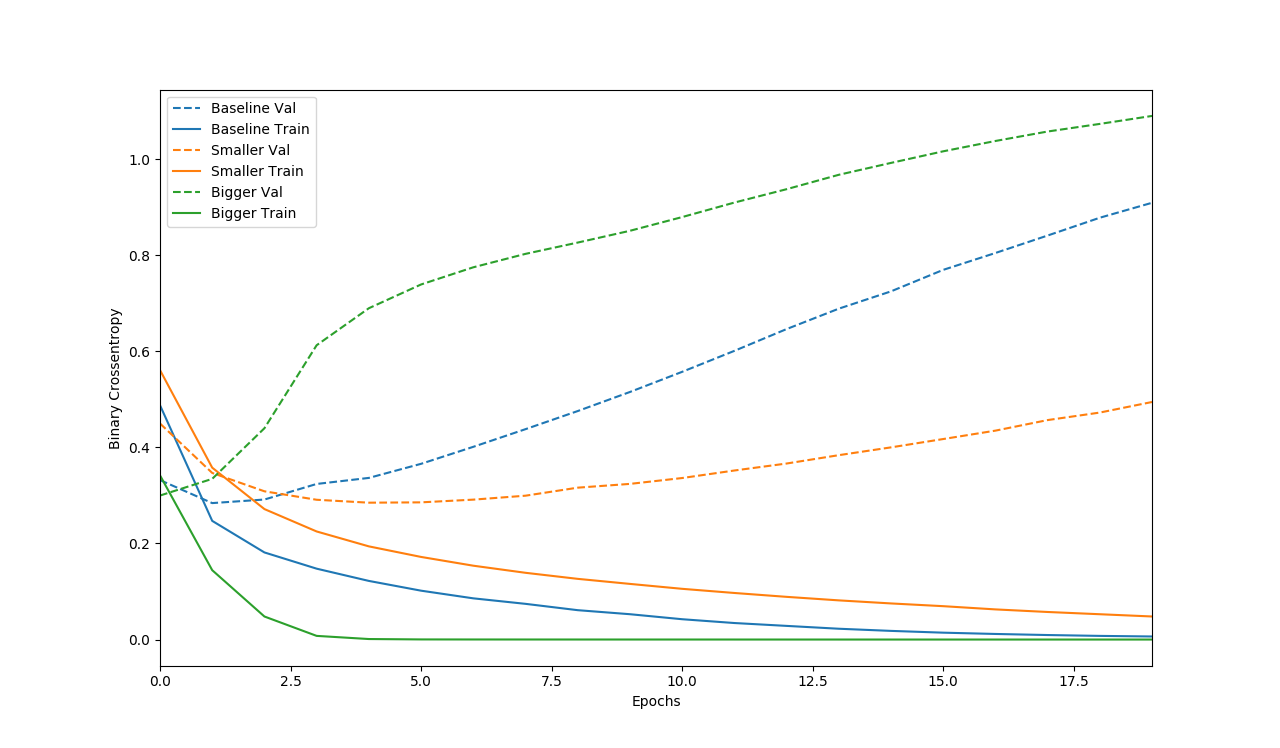#### 优化过拟合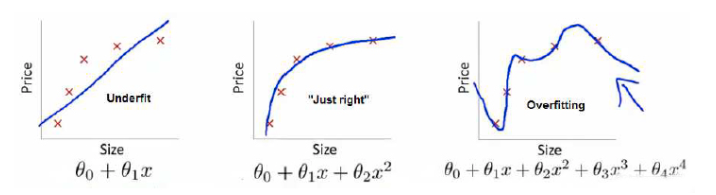如果一个模型产生过拟合，那这个模型的总体效果就可能是一个非常复杂的非线性方程。方程非常努力的学习所有“可见”数据，导致了复杂的权重值，使得曲线弯来弯去，变得极为复杂。多层网络更加剧了这种复杂度，最终的复杂曲线绕开了可行的区域，只对局部的可见数据有效，对于实际数据命中率低。所以从我们程序跑的结果图来看，也是越复杂的网络模型，过拟合现象反而越严重。

#### 增加权重的规范化

``````# 构建一个L2规范化的模型
l2_model = keras.models.Sequential([
keras.layers.Dense(16, kernel_regularizer=keras.regularizers.l2(0.001),
activation='relu', input_shape=(NUM_WORDS,)),
keras.layers.Dense(16, kernel_regularizer=keras.regularizers.l2(0.001),
activation='relu'),
keras.layers.Dense(1, activation='sigmoid')
])

loss='binary_crossentropy',
metrics=['accuracy', 'binary_crossentropy'])

l2_model_history = l2_model.fit(train_data, train_labels,
epochs=20,
batch_size=512,
validation_data=(test_data, test_labels),
verbose=2)
``````

#### 添加DropOut

DropOut是我们在上个系列中已经讲过的方法，应用的很广泛也非常有效。

``````
dpt_model = keras.models.Sequential([
keras.layers.Dense(16, activation='relu', input_shape=(NUM_WORDS,)),
keras.layers.Dropout(0.5),
keras.layers.Dense(16, activation='relu'),
keras.layers.Dropout(0.5),
keras.layers.Dense(1, activation='sigmoid')
])

loss='binary_crossentropy',
metrics=['accuracy','binary_crossentropy'])

dpt_model_history = dpt_model.fit(train_data, train_labels,
epochs=20,
batch_size=512,
validation_data=(test_data, test_labels),
verbose=2)
....
# 最后的绘图函数不变，绘图语句修改如下：
plot_history([
('baseline', baseline_history),
('l2', l2_model_history),
('dropout', dpt_model_history)])
``````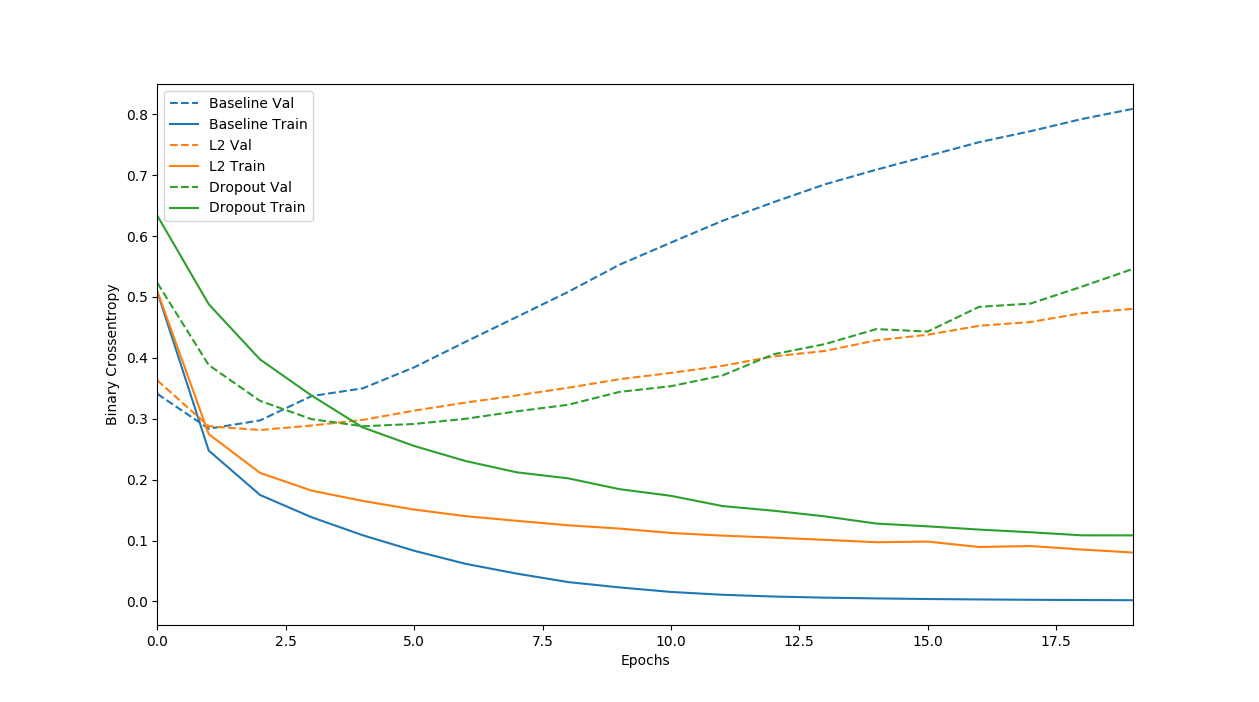（待续…）